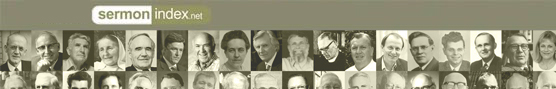Promoting Genuine Biblical Revival.
Sermon Podcast | Audio | VideoSermonIndex.net : Christian Books : PART FIFTH.--THE KINGDOM AND PEOPLE OF JUDAH

Hurlbuts Bible Lessons by Rev. Jesse Lyman Hurlbut

### PART FIFTH.--THE KINGDOM AND PEOPLE OF JUDAH

Lesson XXXVIII. The Early Kings of Judah.

(Tell Stories 1, 2 and 3 in Part Fifth.)

=1.= Where was the kingdom of J[=u]´dah? =West of the Dead Sea.=

=2.= What was its chief city? =J[+e]-r[u:]´s[+a]-l[)e]m.=

=3.= How many kings reigned over the kingdom of J[=u]´dah? =Nineteen kings and one queen.=

=4.= To what family did all these kings belong? =To the family of D[=a]´vid.=

=5.= Who was the first king of J[=u]´dah? =R[=e]-ho-b[=o]´am.=

=6.= Who was the greatest and strongest of the kings of J[=u]´dah? =J[+e]-h[)o]sh´a-ph[)a]t=.

=7.= What wicked woman made herself queen and ruled the land? =[)A]th-a-l[=i]´ah.=

=8.= What little boy was crowned king after [)A]th-a-l[=i]´ah? =J[=o]´[)a]sh.=

=9.= What king became a leper? =[)U]z-z[=i]´ah.=

=10.= What great prophet lived in J[=u]´dah at that time and saw the Lord in the temple? =[=I]-[s+][=a]´iah.=

Lesson XXXIX. The Later Kings of Judah.

(Tell Stories 4, 5 and 6 in Part Fifth.)

=1.= Who was the best of all the kings of J[=u]´dah? =H =3.= What great work did [)E]z´r[.a] do? =He brought together the books of the Old Testament.=

=4.= What did [)E]z´r[.a] do at J[+e]-r[u:]´s[+a]-l[)e]m? =He taught the people to obey God's law.=

=5.= What other good man came while [)E]z´r[.a] was at J

<<  Contents  >>